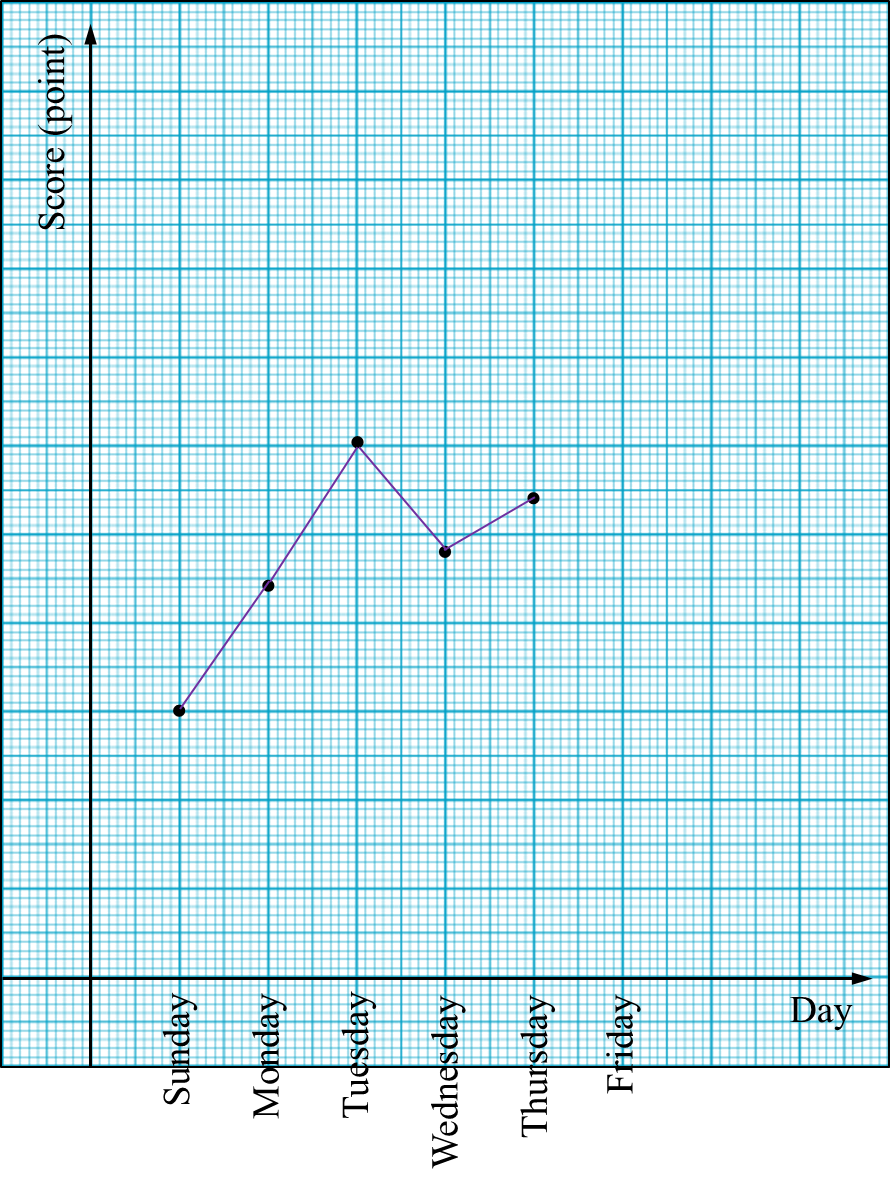# PT3 Mathematics 2017, Question 10

Question 10 (a):
Diagram 10.1 shows a hexagon.Based on the Diagram, complete Table 10.1 in the answer space with the correct answer.Solution:Question 10 (b):
Diagram 10.2 shows a line graph for Leela’s scores obtained at a netball practice. Score on Friday is not shown.Leela’s score on Sunday is 30.
(i) Leela will be rewarded by her coach if her score reaches more than 55 points.
State the day that Leela gets the reward.
(ii) The mean score from Sunday to Friday is 45.
Calculate Leela’s score on Friday.

Solution:
(i)
Tuesday

(ii)

Question 10 (c):
A group of students from SMK Kemayan has produced a coin bank in the shape of a right circular cylinder from pieces of aluminium consisting of a rectangle and two circles of the same size as shown in Diagram 10.3.The height of the coin bank is 20 cm.
Calculate the volume, in cm3 of the coin bank.

Solution: